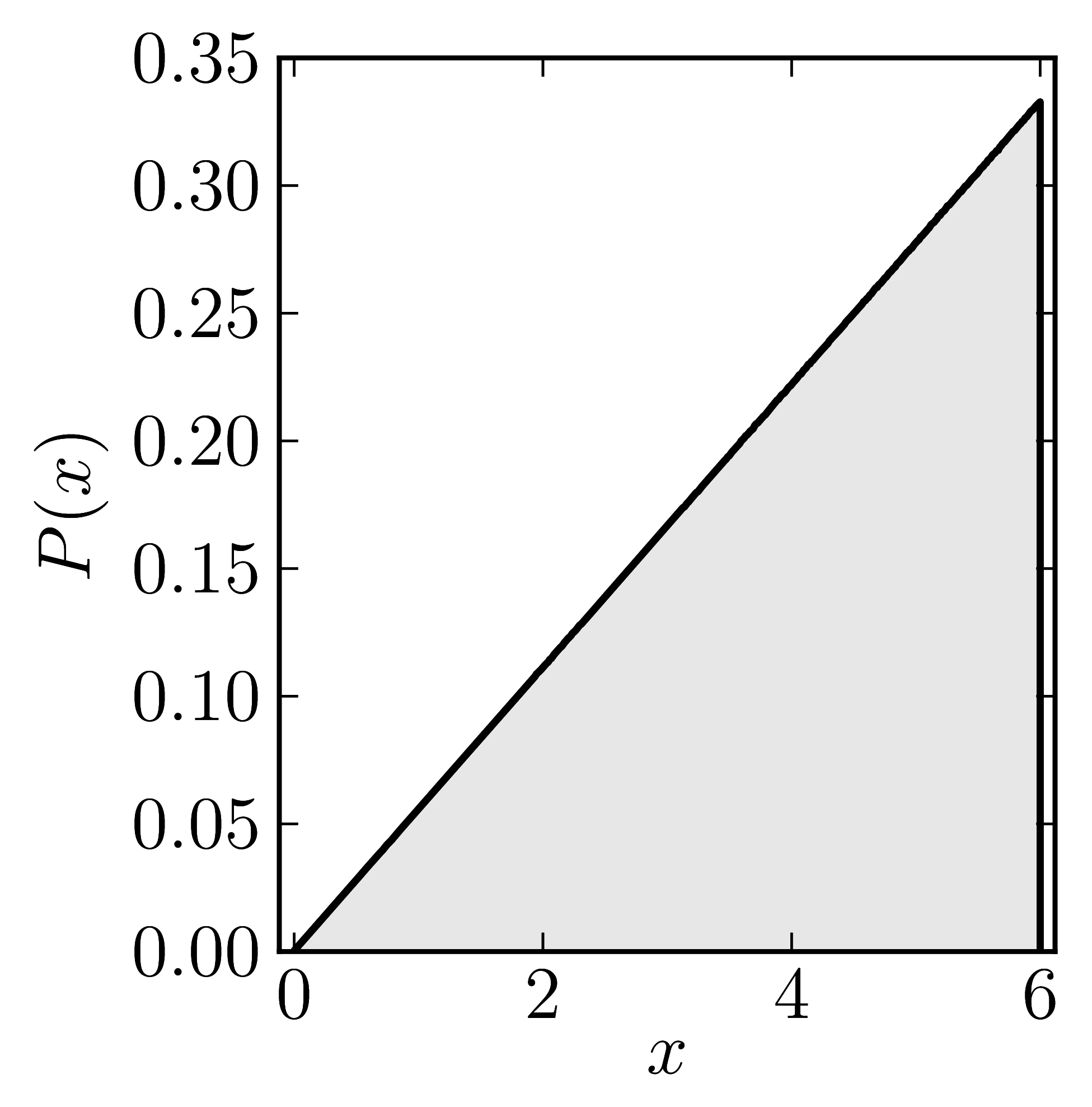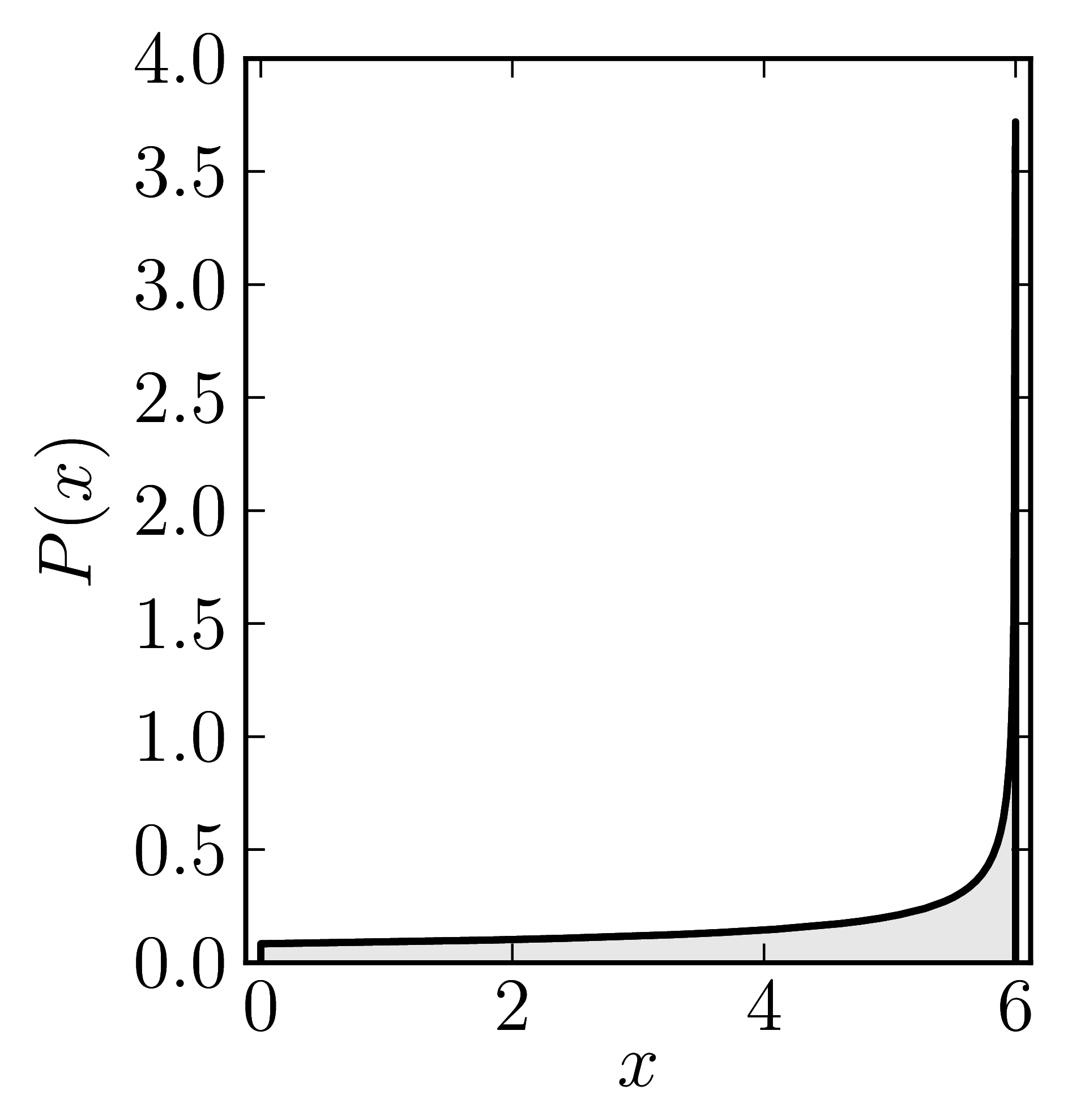# Hermitian matrices

In the case of Hermitian matrices the numerical shadow is a one dimensional distribution.

## Diagonal matrices

### Example 1

The matrix is $\mathrm{diag}(0,3,6)$

Standard numerical shadow with respect to complex states Numerical shadow with respect to real states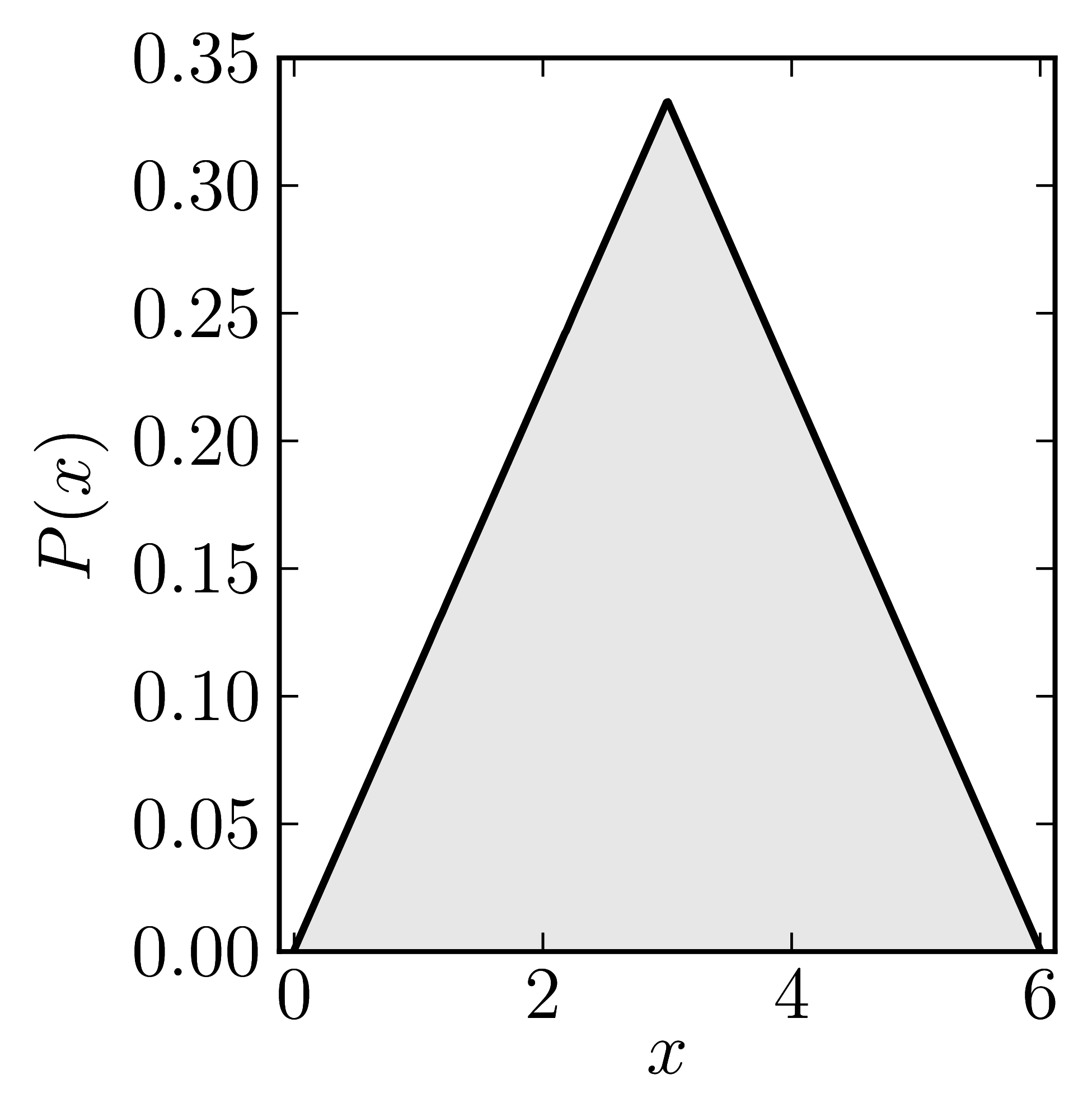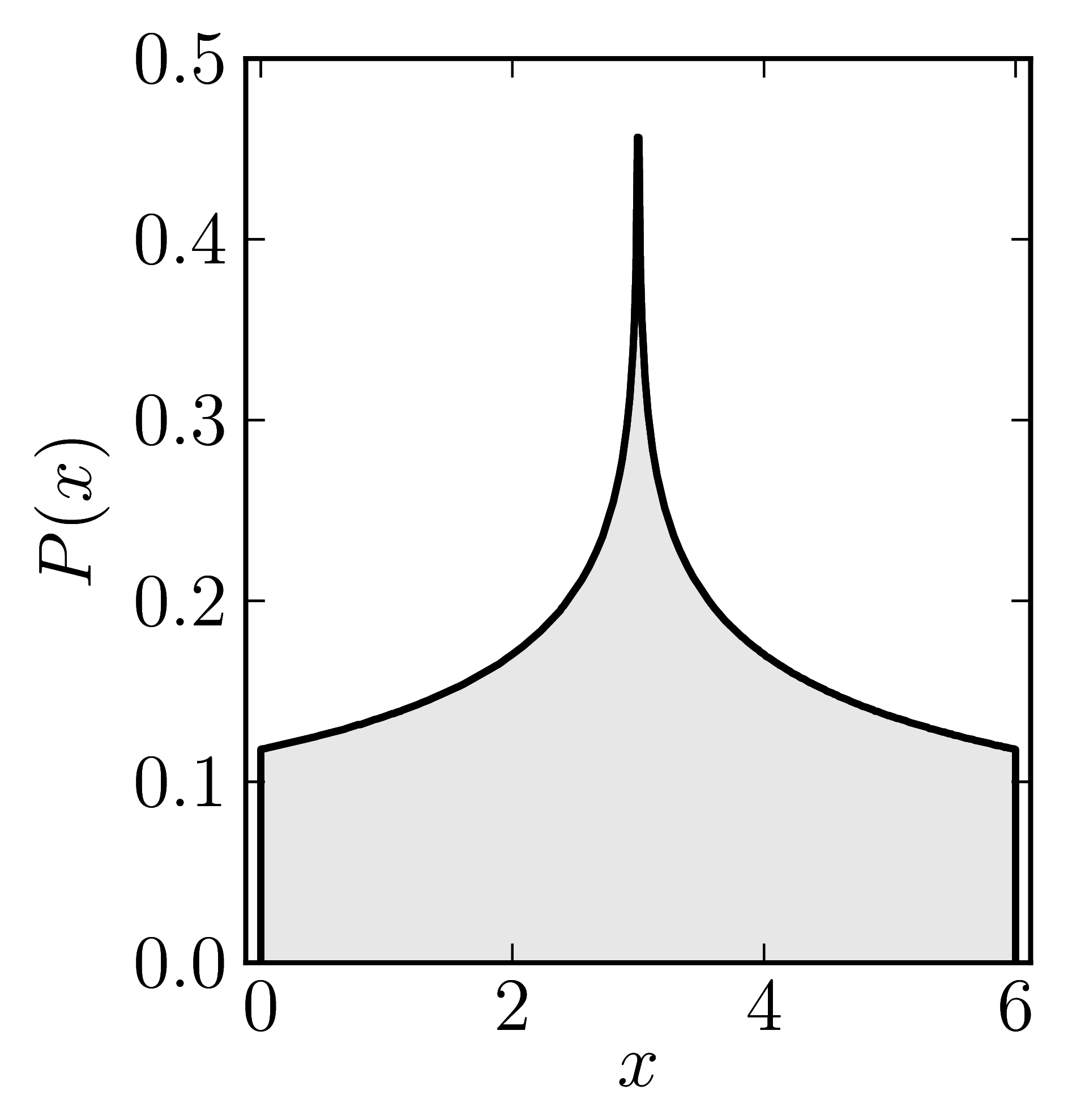### Example 2

The matrix is $\mathrm{diag}(0,4,6)$

Standard numerical shadow with respect to complex states Numerical shadow with respect to real states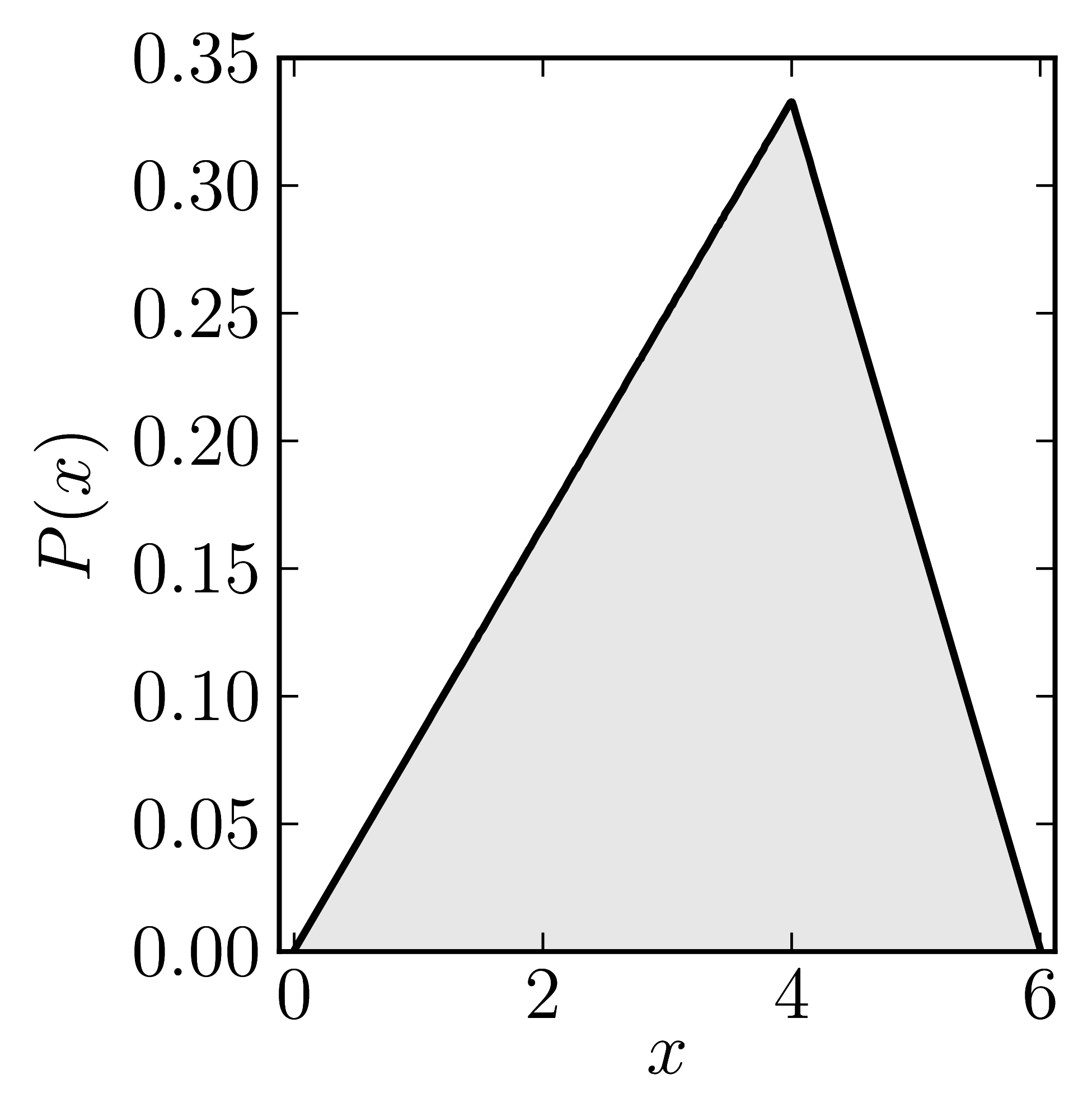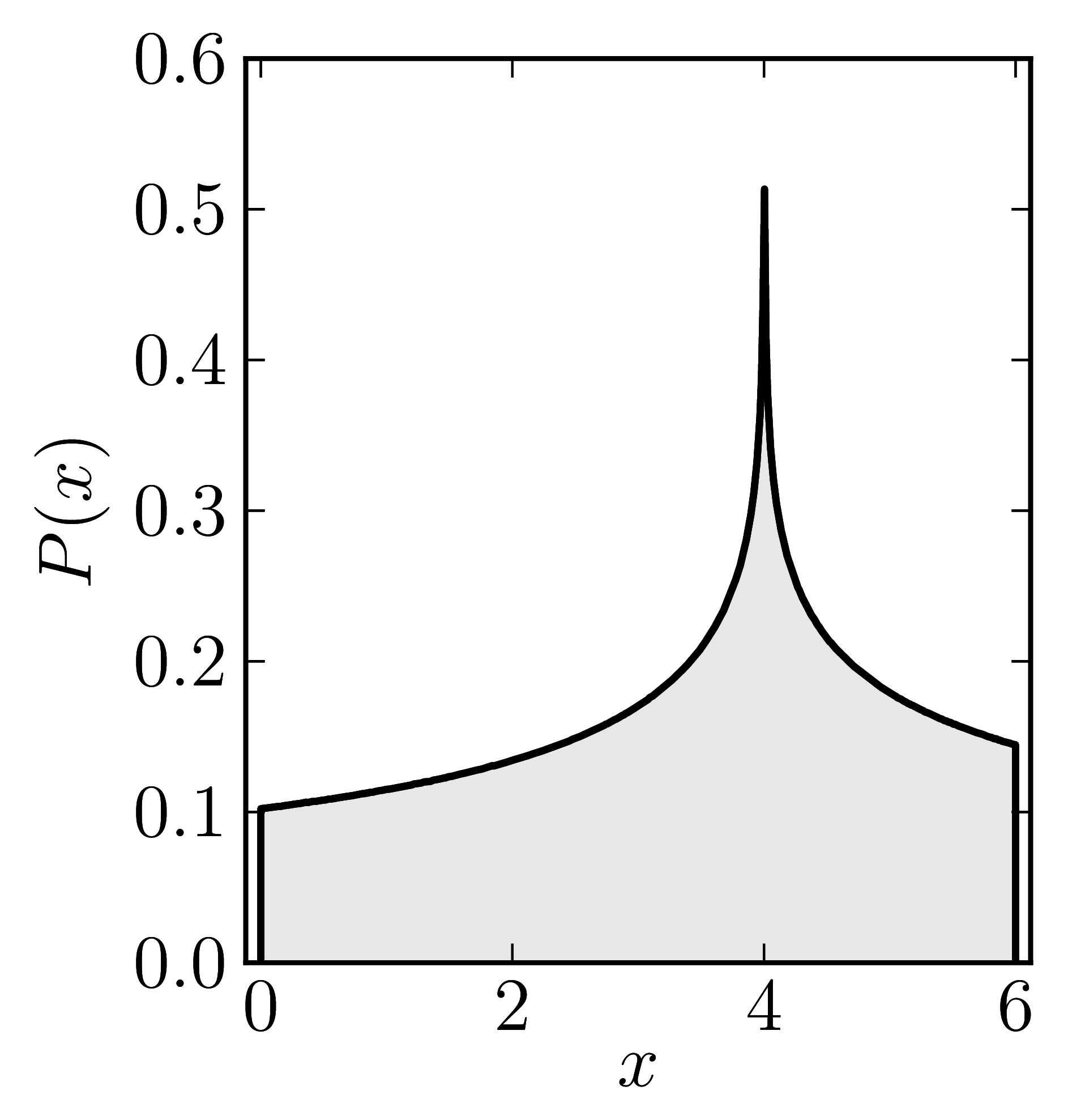### Example 3

The matrix is $\mathrm{diag}(0,5,6)$

Standard numerical shadow with respect to complex states Numerical shadow with respect to real states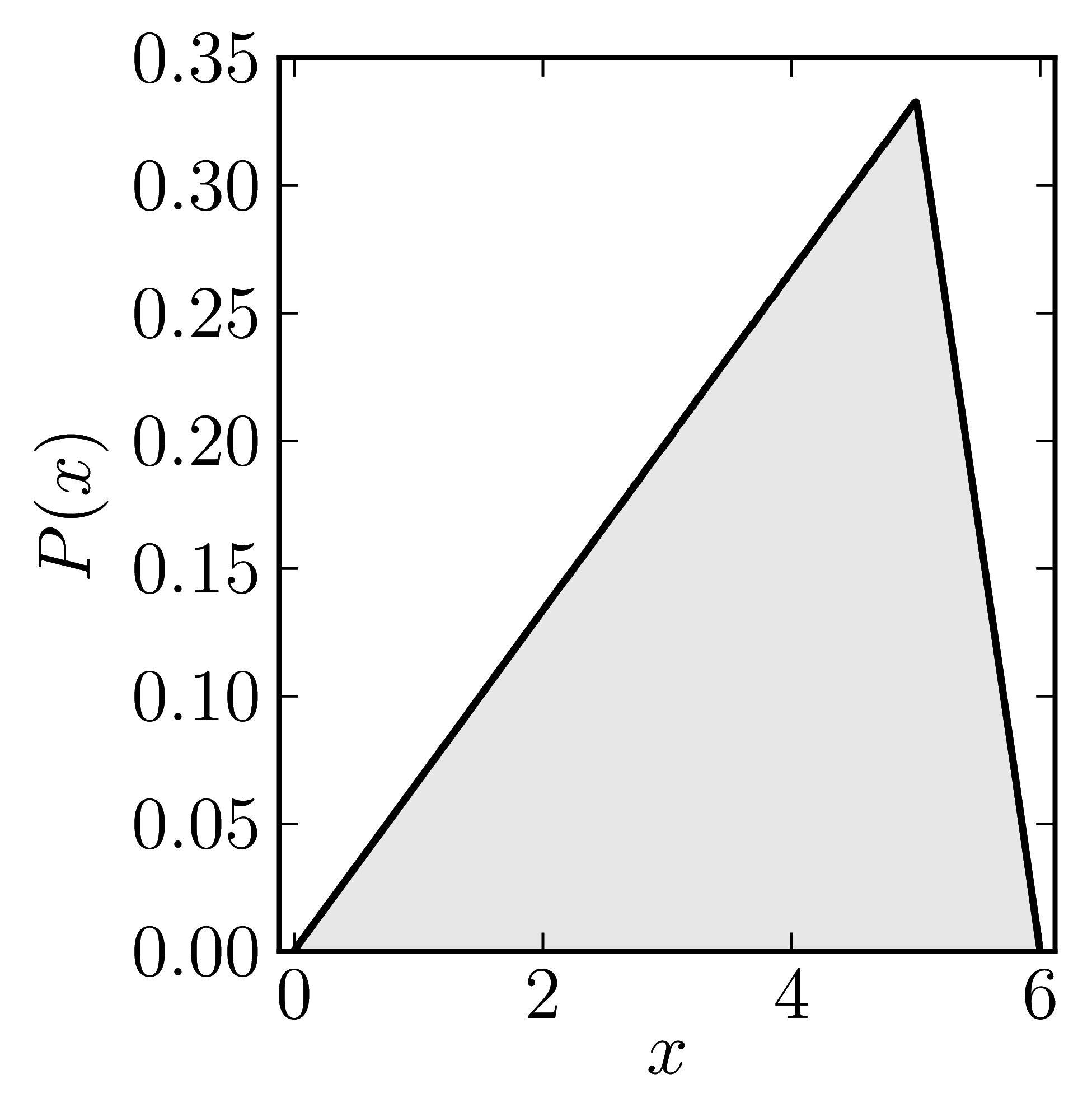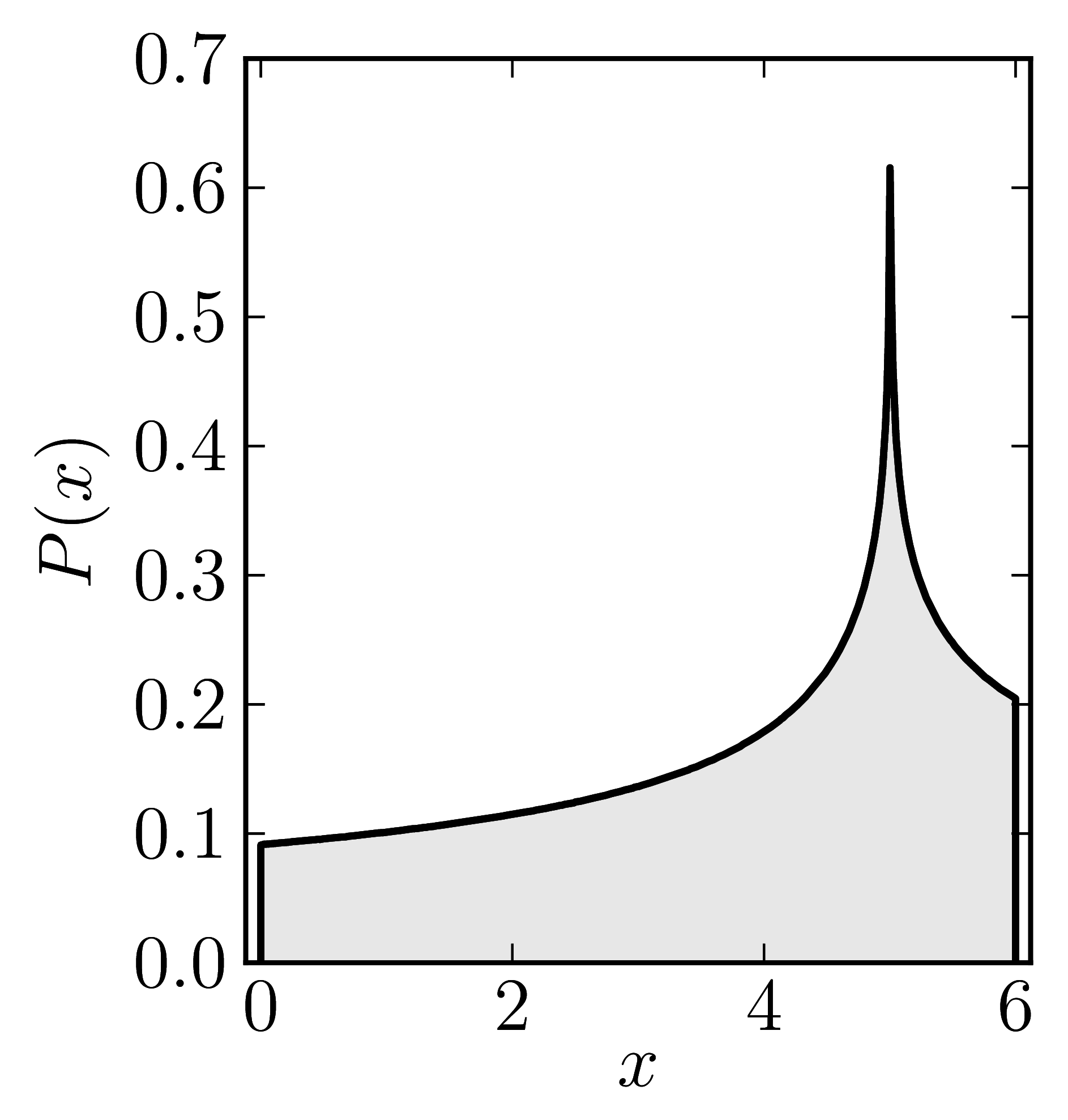### Example 4

The matrix is $\mathrm{diag}(0,6,6)$

Standard numerical shadow with respect to complex states Numerical shadow with respect to real states